# Anderson-Darling statistic

In the goodness-of-fit problem (cf. Goodness-of-fit test) one wants to test whether the distribution function of a random variablebelongs to a given set of distribution functions. In the simplest case this set consists of one completely specified (continuous) distribution function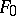, say. A well-known class of test statistics for this testing problem is the class of EDF statistics, thus called since they measure the discrepancy between the empirical distribution function and. The empirical distribution functionis a non-parametric statistical estimator of the true distribution function based on a sample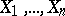. The weighted Cramér–von Mises statistics form a subclass of the EDF statistics. They are defined by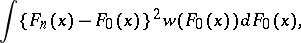where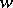is a non-negative weight function. The weight function is often used to put extra weight in the tails of the distribution, since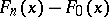is close to zero at the tails and some form of relative error is more attractive.

A particular member of this subclass is the Anderson–Darling statistic, see [a1], [a2], obtained by taking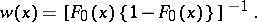To calculate the Anderson–Darling statistic one may use the following formula: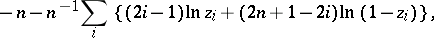with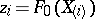and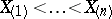the ordered sample.

It turns out, cf. [a7] and references therein, that the Anderson–Darling test is locally asymptotically optimal in the sense of Bahadur under logistic alternatives (cf. Bahadur efficiency). Moreover, under normal alternatives its local Bahadur efficiency is, and hence the test is close to optimal.

In practice, it is of more interest to test whether the distribution function ofbelongs to a class of distribution functions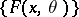indexed by a nuisance parameter, as, for instance, the class of normal, exponential, or logistic distributions. The Anderson–Darling statistic is now obtained by replacing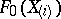byin calculating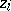, where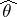is an estimator of. Often, the maximum-likelihood estimator (cf. also Maximum-likelihood method) is used, but see [a5] for a discussion on the use of other estimators.

Simulation results, cf. [a3], [a4], [a5], [a6] and references therein, show that the Anderson–Darling test performs well for testing normality (cf. Normal distribution), and is a reasonable test for testing exponentiality and in many other testing problems.

How to Cite This Entry:
Anderson-Darling statistic. Encyclopedia of Mathematics. URL: http://encyclopediaofmath.org/index.php?title=Anderson-Darling_statistic&oldid=22021
This article was adapted from an original article by W.C.M. Kallenberg (originator), which appeared in Encyclopedia of Mathematics - ISBN 1402006098. See original article